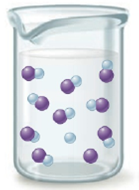Chapter 14, Problem 153AE

Chapter
Section
Textbook Problem

# The following illustration displays the relative number of species when an acid, HA, is added to water.a. Is HA a weak or strong acid? How can you tell?b. Using the relative numbers given in the illustration, determine the value for Ka and the percent dissociation of the acid. Assume the initial acid concentration is 0.20 M.

(a)

Interpretation Introduction

Interpretation: The given acid HA strong or weak, value of Ka for HA upon addition to water, percentage dissociation of HA is to be stated.

Concept introduction: Strong acids dissociate completely and rapidly when dissolved in an aqueous solution and has very large value of Ka while the weak acids dissociates partially and slowly in aqueous solution and has very low value of Ka .

Strong acids have high value of percentage dissociation while weak acids have low value of percentage dissociation.

To determine: The given acid HA is strong or weak.

Explanation

Explanation

The given illustration is,

Figure 1

The given acid HA

(b)

Interpretation Introduction

Interpretation: The given acid HA strong or weak, value of Ka for HA upon addition to water, percentage dissociation of HA is to be stated.

Concept introduction: Strong acids dissociate completely and rapidly when dissolved in an aqueous solution and has very large value of Ka while the weak acids dissociates partially and slowly in aqueous solution and has very low value of Ka .

Strong acids have high value of percentage dissociation while weak acids have low value of percentage dissociation.

To determine: The value of Ka for HA upon addition to water, percentage dissociation of HA .

### Still sussing out bartleby?

Check out a sample textbook solution.

See a sample solution

#### The Solution to Your Study Problems

Bartleby provides explanations to thousands of textbook problems written by our experts, many with advanced degrees!

Get Started